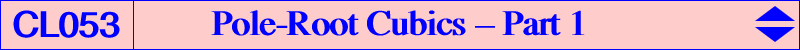Let us consider a non-pivotal cubic nK0(Ω,P) with pole Ω = (p:q:r) and root R = (u:v:w). nK0 is a circular cubic if and only if – either its root R is the transform of its pole Ω under the mapping ΩRc : Ω -> R, where R = b^2c^2p(p+q+r)-(a^4qr+b^4pr+c^4pq) : : , on the line G-tgΩ and on the line passing through the K-Hirst inverse of Ω and the barycentric product of X(523) and the infinite point of the trilinear polar of tgΩ. – or its pole Ω is the transform of its root R under the mapping RΩc : R -> Ω, where Ω = a^2(∑a^2vw)- 2(∑SA u) a^2u : : , on the line K-gtR and on the line passing through PΩc(R) and the gtR–isoconjugate of the trilinear pole of the line R-X(523). These two mappings have the same fixed points namely A, B, C and X(1989), the barycentric product of the Fermat points X(13) and X(14). Each mapping has three singular points : – for RΩc : G, O3, O4, these latter two points are the intersections of the circumcircle with the orthic axis and their barycentric product is X(25), the pole of the orthic axis in the circumcircle, – for ΩRc : K, K1, K2, these latter two points are the infinite points of the circum-conic with perspector X(32) and their barycentric product is X(32). These four points O3, O4, K1, K2 are real when ABC is obtusangle. *** ΩRc maps any point on the line at infinity onto G and RΩc maps any point on the orthic axis onto K. In other words, – there are infinitely many circular nK0s with root G and pole on the line at infinity but these cubics always decompose into the line at infinity and the circumconic which is its isoconjugate, – and infinitely many circular isogonal nK0s with root on the orthic axis with singular focus on the circumcircle and on the cubic hence these are van Rees focal cubics. See Table 4 and glossary. From this, we see that ΩRc transforms a line (L) into a conic (C) passing through G, O3, O4 and RΩc transforms (L) into a conic (C') passing through K, K1, K2. These two correspondences lead to two families Kc1(Q) and Kc2(Q) of interesting cubics when we look at the intersections of a line passing through a fixed point Q and (C) or (C'). This has to be compared with the definition of pivotal isocubics although the two mappings above are not involutions but simply reciprocal transformations. See also CL051. Note that, for a given point Q, the pencil generated by Kc1(Q) and Kc2(Q) contains the pivotal cubic pK(X1989 x Q, X1989) i.e. the pK with pivot X(1989) and isopivot Q. *** The cubics Kc1(Q) These are the cubics Kc1(Q) obtained when we intersect the line (L) and the conic (C). For a given point Q, the cubic Kc1(Q) is a circum-cubic that also contains G, X(1989), O3, O4, Q, ΩRc(Q) and O3O4 /\ KQ. Its meets the sidelines of ABC at U, V, W. This family of cubics contains : – K0s if and only if Q lies on the Euler line and, in this case, it contains X(403), – cubics such that ABC and UVW are perspective when Q lies on a circumcubic through X(3), X(4), X(5), X(49), X(54), X(1147). This gives three pKs namely K489 = Kc1(X3), K490 = Kc1(X4) and K491 = Kc1(X5). The isogonal transform of K491 is K500 = pK(X50, X1993). – cubics such that U, V, W are collinear when Q lies on a circumcubic through X(6), X(110) giving two decomposed cubics, X(184) giving K493 and X(523) giving nK(X1989, X265, X2). The isogonal transform of K493 is the remarkable cubic K494. – one circular decomposed cubic Kc1(X110) already mentioned and one equilateral cubic for a rather complicated Q, – K+ cubics for Q on a cubic without any known center, – nodal cubics when Q = G giving K492 and Q = X(1989).*** The cubics Kc2(Q) These are the cubics Kc2(Q) obtained when we intersect the line (L) and the conic (C'). For a given point Q, the cubic Kc2(Q) is a circum-cubic that also contains K, X(1989), K1, K2, Q, RΩc(Q) and the infinite point of KQ. Its meets the sidelines of ABC at U, V, W. This family of cubics contains : – K0s if and only if Q lies on the Euler line and, in this case, it contains X(25) and X(30), – cubics such that ABC and UVW are perspective when Q lies on the Napoleon cubic K005. This gives three pKs namely K495 = Kc2(X3), K496 = Kc2(X4) and K497 = Kc2(X5). The isogonal transform of K497 is K499 = pK(X323, X69). – cubics such that U, V, W are collinear when Q lies on a circumcubic through X(2), X(110) giving two decomposed cubics, X(523) giving K396 and X(6) giving the nodal cubic cK(#X6, X523) = K381. – one circular decomposed cubic Kc2(G) already mentioned and one equilateral cubic also Kc2(G), – K+ cubics for Q on a cubic without any known center apart G, – nodal cubics when Q = K giving K381 and Q = X(1989). K498 = Kc2(X1) is also a remarkable cubic.